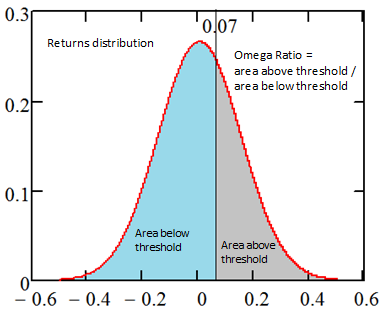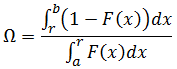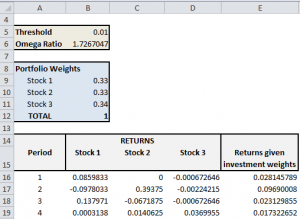# Asset Allocation by Maximizing the Omega Ratio of a Portfolio

This Excel spreadsheet finds the investment weights that maximize the Omega Ratio of a portfolio.  Under realistic conditions, this requires non-convex global optimizers – Excel’s optimizers are not robust enough. So consider the simplified problem in this spreadsheet as a learning exercise.

Realistic problems, which include short selling, a larger investment universe and complex business constraints, require better optimizers. For example, this paper uses NAG routines to optimize the Omega Ratio of a three- and ten-asset portfolio (the data for the spreadsheet is taken from the simpler case).

## The Omega Ratio

Traditional performance benchmarks (like the Sharpe Ratio or Sortino Ratio) approximate the returns distribution with the mean and the standard deviation. This implicitly assumes that the returns are normally distributed, and discards effects like skew and kurtosis.

The Omega Ratio, however, captures all the information in the returns distribution.  It divides the returns distribution into two parts; one part above a threshold and one part below a threshold.The Omega Ratio is simply the area above the threshold divided by the area below the threshold, as described by this equation.In taking this approach, the Omega Ratio models the effect of the disastrous high-impact, low-probability events that are not predicted by a normal distribution.

The Omega Ratio is increasingly being used to benchmark the performance of hedge funds, and other non-traditional investments. These often have an asymmetric returns distribution with significant skew and kurtosis.

Optimizing the Omega Ratio of a portfolio is very different  to traditional mean-variance optimization. The latter balances the effective return of a portfolio against its variance (effectively picking the portfolio with the highest Sharpe Ratio).

Asset allocation by maximizing the Omega Ratio of a portfolio can give higher volatility than mean-variance optimization, but reduces the impact of tail-risk.

## Optimize the Omega Ratio of a Portfolio

This paper uses NAG optimizers to maximize the Omega Ratio of a portfolio of three stocks (as well as a more complex case).  This Excel spreadsheet will reproduce the results of the simpler problem.

An open-source tool was used to digitize the historical returns plots from the paper (Figures 5, 6 and 7), and import the numerical data into an Excel spreadsheet.  This gave 50 returns for each of the three investments.

The portfolio return is the weighted return of the three individual returns.  The Omega Ratio is calculated from this Excel formula (E16:E65 contains the portfolio return, and B5 contains the threshold)

{=SUM(IF(E16:E65 > B5, E16:E65 – B5,””))/-SUM(IF(E16:E65< B5, E16:E65-B5,””))}

It’s a matrix formula so it’s entered with CTRL+SHIFT+ENTER.  See the picture below for an illustration of the spreadsheet.Excel’s Solver can then be used to

• Maximize the Omega Ratio,
• by varying the stock weights,
• subject to the constraints that each weight is between 0 and 1, and the weights add to 1

This models a long-only strategy with no short selling.  See the Solver settings in the picture below.

The spreadsheet employs the standard GRG Nonlinear optimizer with the default tolerances. After optimization, the maximum Omega Ratio is 2.56, with the following investment weights.

• Stock 1: 0.454
• Stock 2: 0.546
• Stock 3: 0

The results match those given in the paper, so we can have some confidence that we’ve taken the right approach.